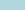### Algebra 1 Worksheets### Algebra 2 Worksheets### Pre-Algebra Worksheets### Elementary Algebra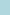### Algebra Word Problems### Intermediate Algebra### Algebra Calculator### Algebra Worksheets# Free Printable Algebra 1 Worksheets - Also Available Online

There are a number of free algebra 1 worksheets for you to download, print, or solve online. The worksheets cover evaluating equations, exponents addition, inequalities, multiplication of exponents, and solving algebra equations in a minimal amount of steps.

Please share our worksheets. Thank you!Evaluate Equations Algebra 1 Worksheet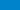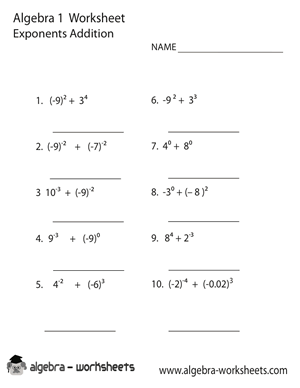Exponents Addition Algebra 1 WorksheetInequalities Algebra 1 Worksheet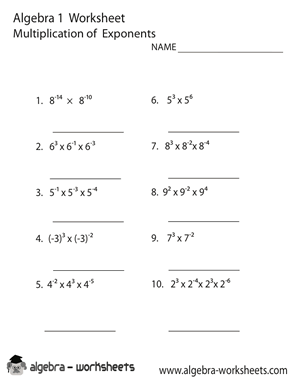Multiplication Exponents Algebra 1 Worksheet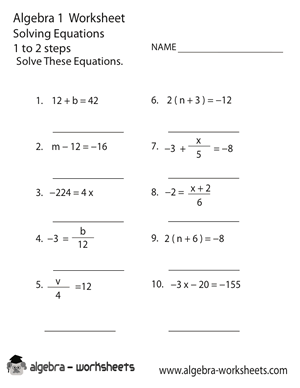Solving Equations Algebra 1 Worksheet## Algebra 1 Worksheets Instructions

Begin by selecting the free algebra worksheet you would like to have. This will take you to the web page of the algebra 1 worksheet. You then have several options. You can print the worksheet, download the corresponding PDF file, or complete the free algebra worksheet online. The online feature works as long as you are using a modern web browser, your iPad or other tablet device. Now you are all ready to start solving algebra equations.

Students will not develop critical thinking and problem solving skills by osmosis. These skills are made. Algebra 1 can help accelerate this process. Students take all of the math knowledge they know to be true and start to expand upon it exponential (no pun intended).

In the beginning of our education, we are taught that numbers are for math and letters are for English. In algebra, we teach students that letters and numbers need to work together so that problems can be solved. If you really look closely, algebra is a lot like life. You grow up thinking things are one way when in fact they are totally different.

### Worksheets Summary

There are two worksheets covering exponents. Exponentiation uses two numbers. One is the base number and the other is the exponent or "power". The exponent is the small number to right of the larger base number. Students can practice adding and multiplying exponents with these printable worksheets.

We have an algebra worksheet that asks students to solve inequalities. This is where they need to show which side in greater than the other side.

Students can practice evaluating a series of equations using the letters x, y, and z to represent numbers. There is also a worksheet where they can solve simple equations in 1 or 2 steps.